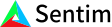1. Bob Anderson
×
IntuitiveML

## Neural Network Caveats (Intuition: Artificial Neural Networks Follow-up)

Jun 2, 2020

A worry that you might have is that our initial function is unable to represent the actual function. For example, if we create an artificial neural network and we try to model the sine function, how can we be sure that the network will learn a close approximation of the function and not just create a linear regression? Well, it’s been proven that as long as you have enough nodes and enough layers, and the function you are using at each of your nodes is non-linear, a neural network can make any function.

However, this doesn’t guarantee that the network is modeling the actual function. It guarantees that it is possible to model that function, but in reality, your neural network is modeling the function over your data set. For instance, if you were trying to model a modified sine function with a collection of data points between [0, 2*pi], it’s entirely possible that the function the network actually finds looks like this, where before this range it always predicts zero and after this range it always predicts one, even though we know the sine function actually looks roughly like this.

This is why it’s better to have as much data as possible: the more data, the more likely the output function is modeling the actual function you are looking for instead of some random function describing your data set.